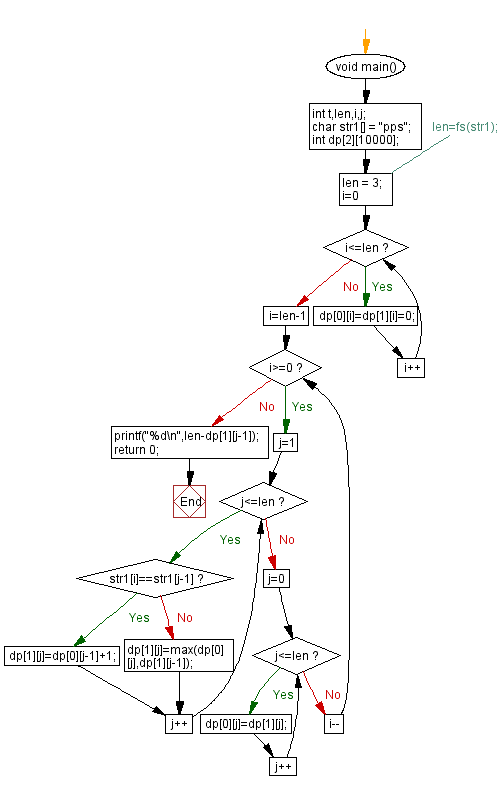﻿ C Program - Minimum number of characters to make a palindrome# C Exercises: Minimum number of characters to make a palindrome

## C Programming Challenges: Exercise-29 with Solution

Write a C program to find the minimum number of characters that must be inserted into a given string with no whitespace characters to make it a palindrome.

Note: For example, if str1 = "pps", we can change the string to "spps", adding only 1 character.

Sample Input: pps
Output: 1

C Code:

``````#include<stdio.h>
#define max(a,b) (a>b?a:b)
int void main()
{
int t,len,i,j;
char str1[] = "pps";
int dp;
//len=fs(str1);
len = 3;
for(i=0;i<=len;i++) dp[i]=dp[i]=0;
for(i=len-1;i>=0;i--)
{
for(j=1;j<=len;j++)
{
if(str1[i]==str1[j-1]) dp[j]=dp[j-1]+1;
else dp[j]=max(dp[j],dp[j-1]);
}
for(j=0;j<=len;j++)
{
dp[j]=dp[j];
}
}
printf("%d\n",len-dp[j-1]);
return 0;
}
``````

Sample Output:

```1
```

Flowchart:## C Programming Code Editor:

Contribute your code and comments through Disqus.

Previous C Programming Exercise: First missing +ve integer in unsorted array.
Next C Programming Exercise: Prime number in strictly ascending decimal digit order.

What is the difficulty level of this exercise?

Test your Programming skills with w3resource's quiz.

﻿